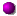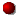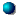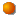# \$B%j%K%"WB@1(B

187P/LINEAR###\$B%W%m%U%#!<%k(B

1999\$BG/\$KH/8+\$5\$l\$??7<~4|WB@1\$@\$,!"

\$BLZ2<0lCK;a\$N7W;;\$G\$O!"(B1952\$BG/0J9_!"6aF|E@5wN%\$O(B3.6\$B!A(B3.7AU\$B\$G!"\$[\$H\$s\$IJQ2=\$7\$F\$\$\$J\$\$!#\$=\$N\$?\$a!"WB@1\$N8wEY\$bK\Mh\$O0BDj\$7\$F\$\$\$k\$O\$:!#(B

1999\$BG/\$N=P8=\$G\$O!"H/8+\$+\$i(B1\$BG/8e\$N(B2000\$BG/\$K\$b!"H/8+;~\$H\$[\$\F1\$8L@\$k\$5\$@\$C\$?\$O\$:\$@\$,!"4QB,\$5\$l\$F\$\$\$J\$\$!#\$h\$C\$F!"(B1999\$BG/\$K%P!<%9%H\$G0l;~E*\$KA}8w\$7\$F\$\$\$?2DG=@-\$b\$"\$k!#(B###\$B2s5"5-O?(B\$BH/8+(B\$B=P8=(B\$B4QB,\$5\$l\$J\$+\$C\$?2s5"(B\$BH/8+A0\$N=P8=(B\$BH/8+A0\$N4QB,\$5\$l\$J\$+\$C\$?2s5"(B\$B>-Mh\$N2s5"(B187P 2018/05/26187P/2007 E3 2008/10/06187P/1999 J5 1999/05/12##### \$B2a5n\$HL\$Mh\$N50F;MWAG\$OLZ2<0lCK;a\$K\$h\$C\$F7W;;\$5\$l\$F\$\$\$k(B(http://www9.ocn.ne.jp/~comet/pcmt/j99j5.htm)\$B!#(B